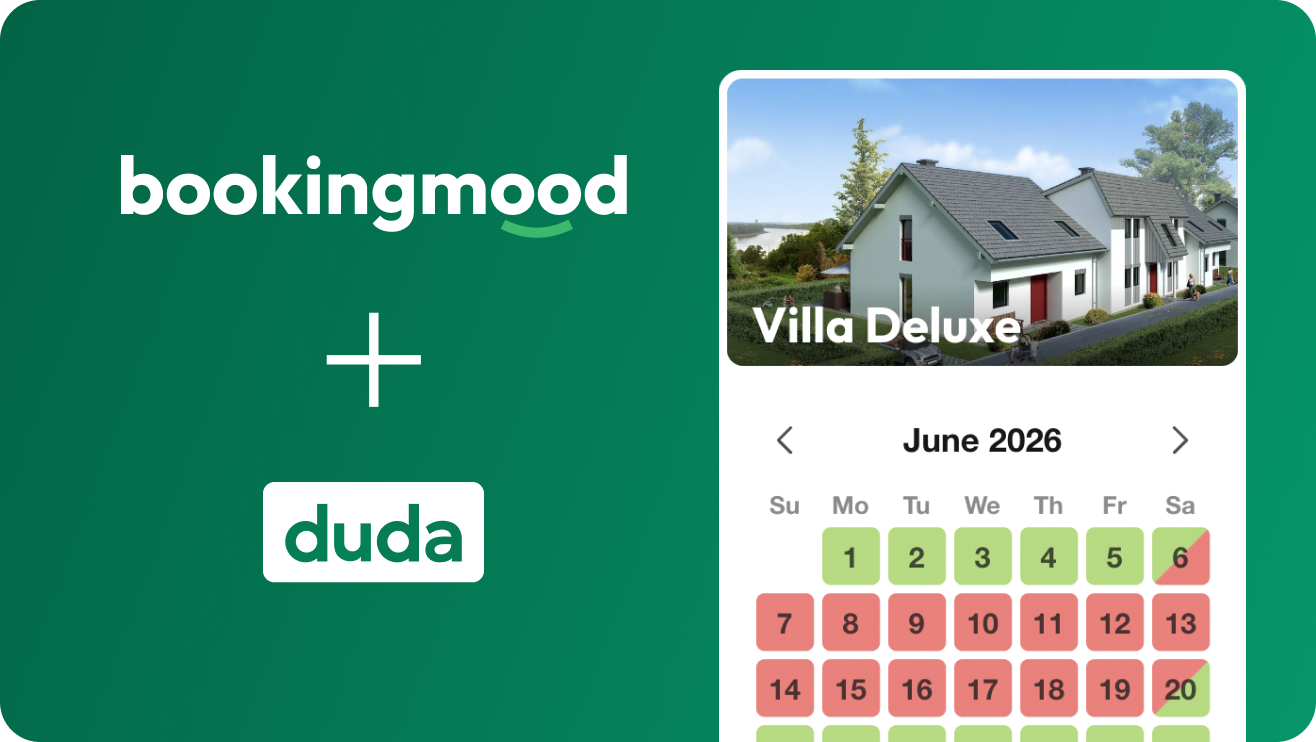# Booking calendar for Duda

## The best booking calendar for your Duda websiteself.__wrap_n=self.__wrap_n||(self.CSS&&CSS.supports("text-wrap","balance")?1:2);self.__wrap_b=(e,t,n)=>{let r=(n=n||document.querySelector(`[data-br="\${e}"]`)).parentElement,i=e=>n.style.maxWidth=e+"px";n.style.maxWidth="";let o=r.clientWidth,a=r.clientHeight,l=o/2-.25,s=o+.5,c;if(o){for(i(l),l=Math.max(n.scrollWidth,l);l+1<s;)i(c=Math.round((l+s)/2)),r.clientHeight===a?s=c:l=c;i(s*t+o*(1-t))}n.__wrap_o||"undefined"!=typeof ResizeObserver&&(n.__wrap_o=new ResizeObserver(()=>{self.__wrap_b(0,+n.dataset.brr,n)})).observe(r)};self.__wrap_n!=1&&self.__wrap_b(":R3aqpamlla:",1)Hey Duda user! Are you looking for a booking calendar to embed on your Duda website? Bookingmood is a simple booking calendar that blends into your Duda website perfectly. It works as simple as Duda. Start showing your availability and accept bookings today!

Embedding Bookingmood in Duda took us just 2 minutes!

View the manual

## Duda and Bookingmood:self.__wrap_n=self.__wrap_n||(self.CSS&&CSS.supports("text-wrap","balance")?1:2);self.__wrap_b=(e,t,n)=>{let r=(n=n||document.querySelector(`[data-br="\${e}"]`)).parentElement,i=e=>n.style.maxWidth=e+"px";n.style.maxWidth="";let o=r.clientWidth,a=r.clientHeight,l=o/2-.25,s=o+.5,c;if(o){for(i(l),l=Math.max(n.scrollWidth,l);l+1<s;)i(c=Math.round((l+s)/2)),r.clientHeight===a?s=c:l=c;i(s*t+o*(1-t))}n.__wrap_o||"undefined"!=typeof ResizeObserver&&(n.__wrap_o=new ResizeObserver(()=>{self.__wrap_b(0,+n.dataset.brr,n)})).observe(r)};self.__wrap_n!=1&&self.__wrap_b(":Rdaqpamlla:",1)

• Enable the option to book directly from your Duda website
• Easy to manage

## Bookingmood is 100% compatible with Dudaself.__wrap_n=self.__wrap_n||(self.CSS&&CSS.supports("text-wrap","balance")?1:2);self.__wrap_b=(e,t,n)=>{let r=(n=n||document.querySelector(`[data-br="\${e}"]`)).parentElement,i=e=>n.style.maxWidth=e+"px";n.style.maxWidth="";let o=r.clientWidth,a=r.clientHeight,l=o/2-.25,s=o+.5,c;if(o){for(i(l),l=Math.max(n.scrollWidth,l);l+1<s;)i(c=Math.round((l+s)/2)),r.clientHeight===a?s=c:l=c;i(s*t+o*(1-t))}n.__wrap_o||"undefined"!=typeof ResizeObserver&&(n.__wrap_o=new ResizeObserver(()=>{self.__wrap_b(0,+n.dataset.brr,n)})).observe(r)};self.__wrap_n!=1&&self.__wrap_b(":Rhaqpamlla:",1)

We tested Bookingmood with Duda and it passed all tests 😀. You just have to embed your Bookingmood widget once. After that is done, every booking you add in Bookingmood will be visible on your Duda site immediately.

View all features